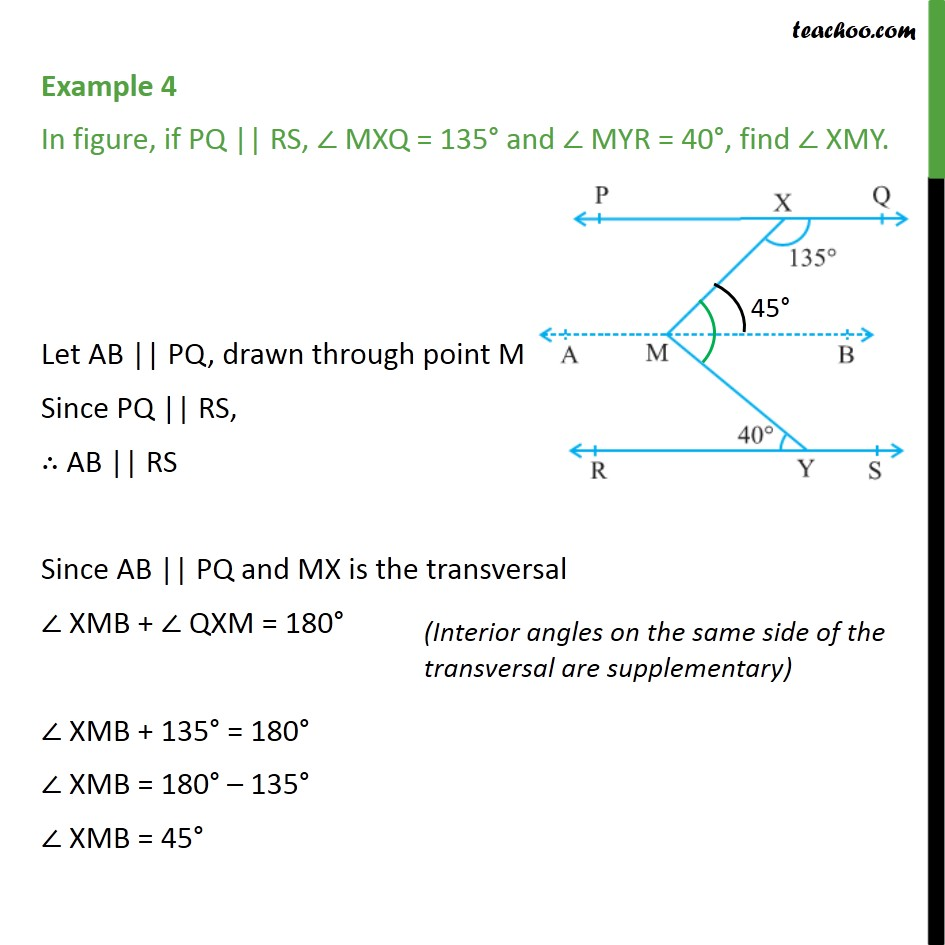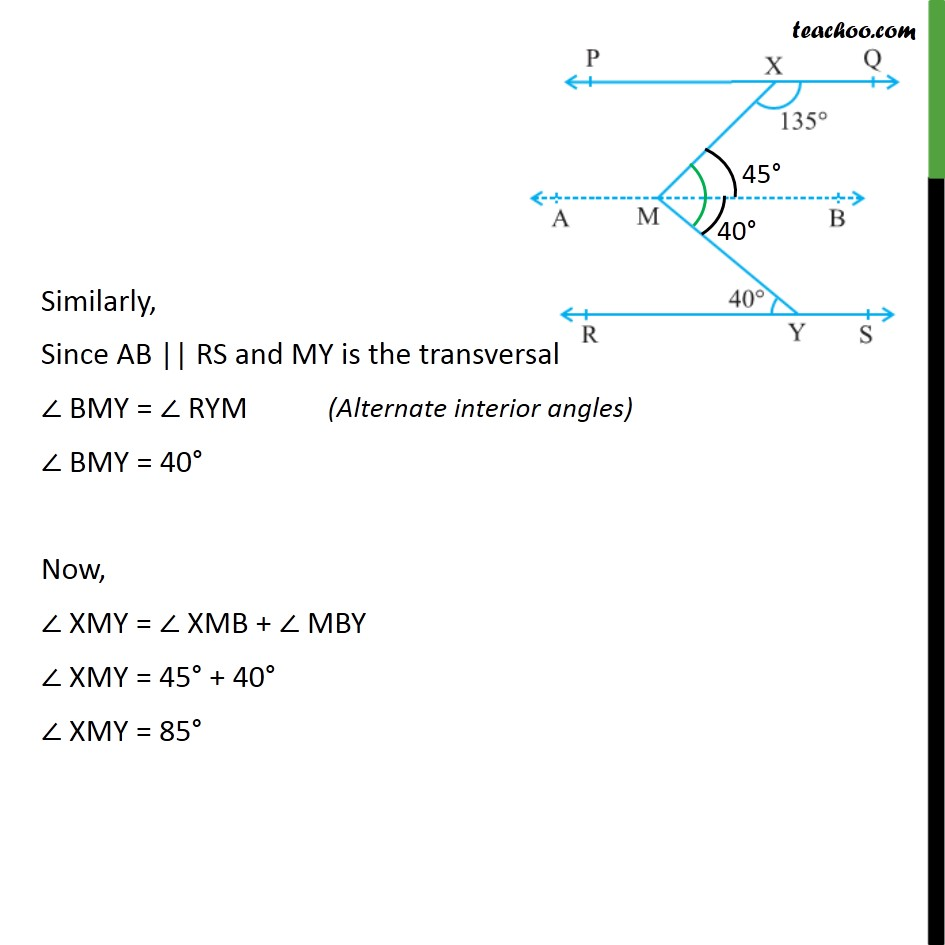Examples

Chapter 6 Class 9 Lines and Angles
Serial order wiseLearn in your speed, with individual attention - Teachoo Maths 1-on-1 Class

### Transcript

Example 4 In figure, if PQ || RS, MXQ = 135 and MYR = 40 , find XMY. Let AB || PQ, drawn through point M Since PQ || RS, AB || RS Since AB || PQ and MX is the transversal XMB + QXM = 180 XMB + 135 = 180 XMB = 180 135 XMB = 45 Similarly, Since AB || RS and MY is the transversal BMY = RYM BMY = 40 Now, XMY = XMB + MBY XMY = 45 + 40 XMY = 85